Courses

# Test: Highway Geometric Design - 3

## 20 Questions MCQ Test Transportation Engineering | Test: Highway Geometric Design - 3

Description
This mock test of Test: Highway Geometric Design - 3 for Civil Engineering (CE) helps you for every Civil Engineering (CE) entrance exam. This contains 20 Multiple Choice Questions for Civil Engineering (CE) Test: Highway Geometric Design - 3 (mcq) to study with solutions a complete question bank. The solved questions answers in this Test: Highway Geometric Design - 3 quiz give you a good mix of easy questions and tough questions. Civil Engineering (CE) students definitely take this Test: Highway Geometric Design - 3 exercise for a better result in the exam. You can find other Test: Highway Geometric Design - 3 extra questions, long questions & short questions for Civil Engineering (CE) on EduRev as well by searching above.
QUESTION: 1

### If an ascending gradient of 1 in 50 meets another ascending gradient of 1 in 30 then the deviation angle is

Solution: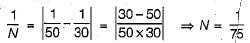QUESTION: 2

Solution:
QUESTION: 3

### Width Of carriage way for a single lane is recommended to be

Solution:
QUESTION: 4

Stopping sight distance is the minimum distance available on a highway which is the

Solution:
QUESTION: 5

The design value of lateral friction coefficient on highway is

Solution:
QUESTION: 6

Camber on highway pavement is provided to take care of

Solution:
QUESTION: 7

In the limiting case of overturning while traversing a simple circular curve by a vehicle, pressure below

Solution:

If the pavement is kept horizontal across the alignment, the pressure on the outer wheels will be higher due to the centrifugal force acting outwards and hence the reaction RB at the outer wheel would be higher. When the limiting equilibrium condition for overturning occurs the pressure at the inner wheels becomes equal to zero and pressure at outer wheels will be maximum.

QUESTION: 8

The tandwidth required across the alignment of road is

Solution:

Right of way is the area of land acquired for the road, along its alignment. The width of this acquired land is known as land width and it depends on the importance of the road and possible future development.

QUESTION: 9

Solution:

Factors which govern the alignment of road are,
1. Obligatory points: These are points either the road alignment has to pass or. not have to pass. '
2. Traffic: The new road to be aligned should keep in view the desired lines, traffic flow patterns and future trends.
3. Geometric Design.
4. Economy.
5. Stability of hill-drainage and geometric standard of hili roads.

QUESTION: 10

Parabolic camber is preferred for

Solution:

The camber is given a parabolic elliptic or straight line shape in the cross section parabolic or elliptic shape is given so that the profile is flat at the middle and steeper towards the edges, which is preferred by fast moving vehicles as the have to frequently cross the crown line during overtaking  operation on a two lane highway.

QUESTION: 11

Stopping sight distance on a national highway at a descending gradient of 2% is

Solution:

Desigmsffeed on national highway,
V = 80 km/hr
SSD = vt+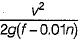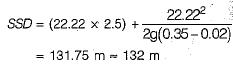QUESTION: 12

Which one of the following pairs is correctly matched? (Notations have their usual meaning)

Solution: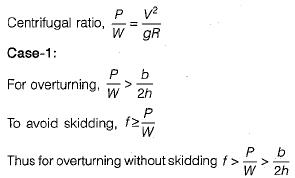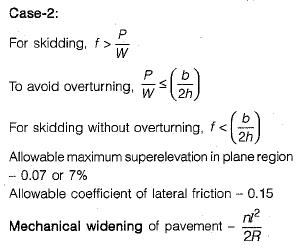QUESTION: 13

A road camber given in the following figure: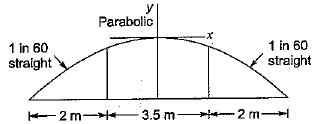For designing this camber the equation to be used is

Solution:
QUESTION: 14

The compensated gradient provided at the curve of radius 60 m with a ruling gradient of 6 percent is:

Solution: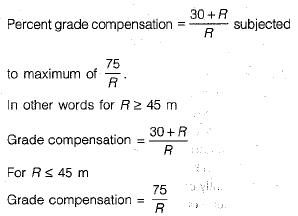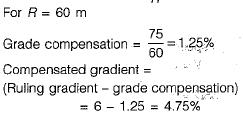QUESTION: 15

if N is the algebraic difference of grades, S is the headlight sight distance in metres, then the transmission length of a valley curve (following standard codes) should roughly be equal to

Solution:

The head light sight distance should be at least equal to SSD. If the vehicles are overtaking then length curve should be: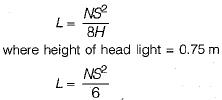QUESTION: 16

The design speed of a highway is 80 km per hour. Assuming other data as per IRC recommendations, which one of the following is the approximate adopted lag distance?

Solution:

Lag distance = 0. 278 Vt
Reaction time t = 2.5 sec
Lag distance = 55.6 m

QUESTION: 17

Consider the following steps involved in the design of super elevation in practice as recommended by IRC :
1. Calculation of the allowable speed for maximum ‘e’ and design value of T
2. Calculation of the super elevation for 75% of the design speed
3. Calculation of the value of ‘e’ and recheck
4. Calculation of the value of ‘f and recheck
The correct sequence of these steps is

Solution:

2 - 3 - 4 - 1
The step given in 1 is an alternative of step given in 4.

QUESTION: 18

In highway geometric design, cumulative speed distribution is drawn and the design in checked at which and the design in checked at which percentile?

Solution:

85th percentile speed is upper limit of speed for regulation and 15th percentile speed is lower limit of speed for regulation.
The 98th percentile speed is the design speed,

QUESTION: 19

Which one of the, following is taken in to consideration while determining overtaking sight distance in a two lane highway

Solution:
QUESTION: 20

Match List-I (Type of road) with List-ll (Recommended camber) and select the correct answer by using the codes given below the lists: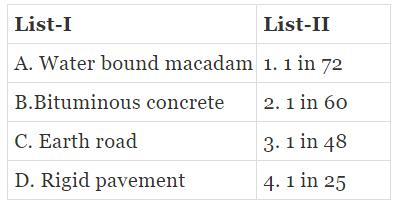Codes: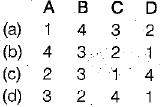Solution: